Like   Tweet   Pin   +1   in

## 2019 Fall Mini dates announced!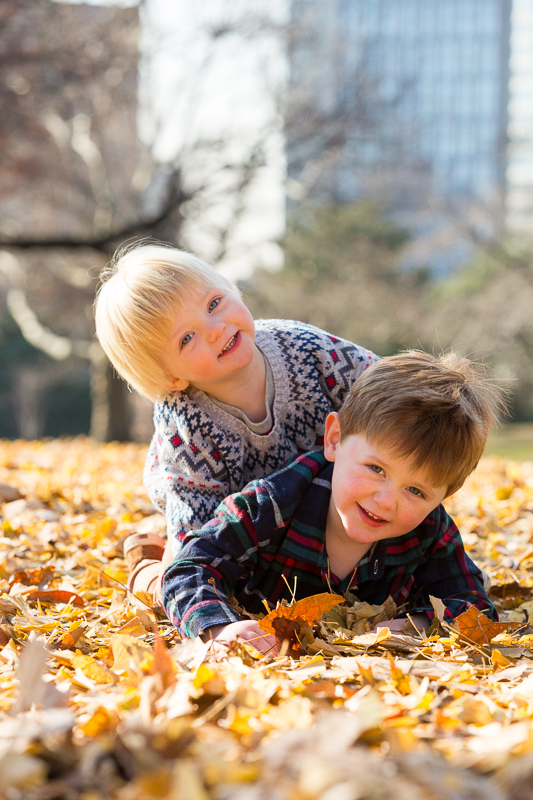Fort Greene Park
 /* styles */ Fall is officially on the way. We just added dates for Central Park and Brooklyn designed to show off fall's colors and your family. A few spots are available with Petra this Sunday and Columbus Day if you want something sooner.
 table div table+table+table+table+table div table{width:100%;padding:0}table div table+table+table+table+table div table img{width:96.23%;padding:0;float:none}table div table+table+table+table+table div table td{width:100%;padding:0 1.88% 18px}/* styles */## The Dates and Locations

 /* styles */
 table div table+table+table+table+table+table+table+table div table{width:100%;padding:0}table div table+table+table+table+table+table+table+table div table img{width:96.23%;padding:0;float:none}table div table+table+table+table+table+table+table+table div table td{width:100%;padding:0 1.88% 18px}/* styles */table div table+table+table+table+table+table+table+table+table+table div table{width:100%;padding:0}table div table+table+table+table+table+table+table+table+table+table div table img{width:96.23%;padding:0;float:none}table div table+table+table+table+table+table+table+table+table+table div table td{width:100%;padding:0 1.88% 18px}/* styles */## The Lowdown

 /* styles */ Petra and Justine each offer a 25' Mini Session Special for either \$225 with Petra or \$275 with Justine. Individual touched up digital files for holiday cards are \$45 each. Upgrade to have the full set of high resolution files (usually 20-40 final images) for an additional \$300 for Petra's session, or \$350 for Justine's session. All sessions include a private viewing gallery online for 30 days. Available add-ons include great options for gifts, books and artwork once you have seen your images.
 table div table+table+table+table+table+table+table+table+table+table+table+table+table div table{width:100%;padding:0}table div table+table+table+table+table+table+table+table+table+table+table+table+table div table img{width:96.23%;padding:0;float:none}table div table+table+table+table+table+table+table+table+table+table+table+table+table div table td{width:100%;padding:0 1.88% 18px}/* styles */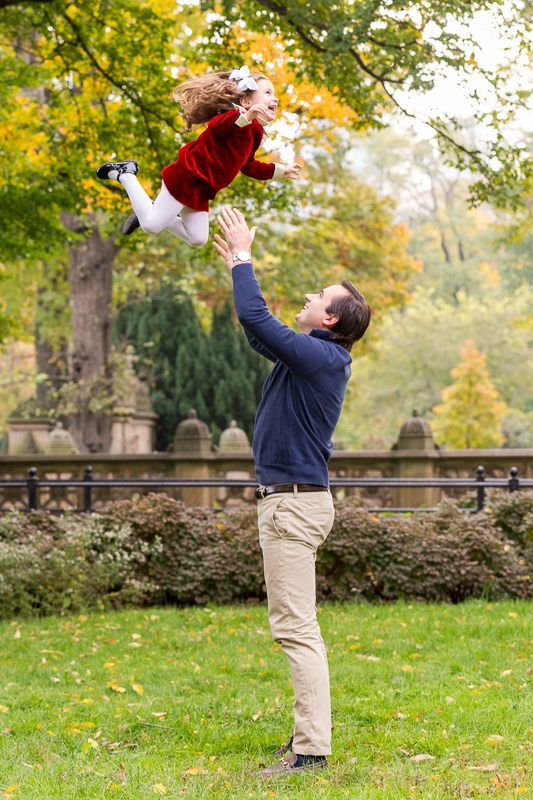Central Park - The MallCentral Park - The Mall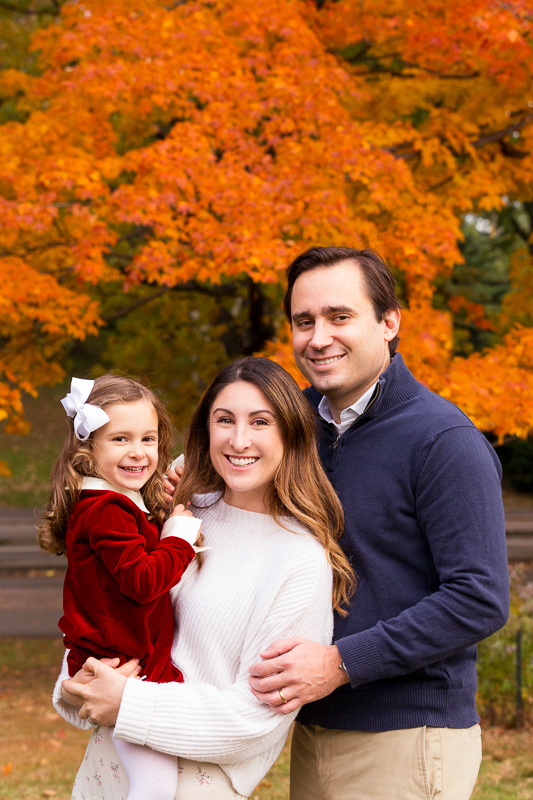Central Park - The Mall

Central Park - The Mall has outstanding foliage, pretty fences, benches and of course the mall itself.

 table div table+table+table+table+table+table+table+table+table+table+table+table+table+table+table+table div table{width:100%;padding:0}table div table+table+table+table+table+table+table+table+table+table+table+table+table+table+table+table div table img{width:96.23%;padding:0;float:none}table div table+table+table+table+table+table+table+table+table+table+table+table+table+table+table+table div table td{width:100%;padding:0 1.88% 18px}/* styles */Teardrop Park
 /* styles */ Teardrop Park in Battery Park City is an intimate park with cool rock walls, photogenic benches and steps, a small interesting playground and a gentle rolling hill.
 table div table+table+table+table+table+table+table+table+table+table+table+table+table+table+table+table+table+table+table div table{width:100%;padding:0}table div table+table+table+table+table+table+table+table+table+table+table+table+table+table+table+table+table+table+table div table img{width:96.23%;padding:0;float:none}table div table+table+table+table+table+table+table+table+table+table+table+table+table+table+table+table+table+table+table div table td{width:100%;padding:0 1.88% 18px}/* styles *//* styles */ Cross a river for incredible Liberty State Park in New Jersey (easy to park a car there) and spend Columbus Day in this historically rich park, stunning "empty sky" memorial, and old trains.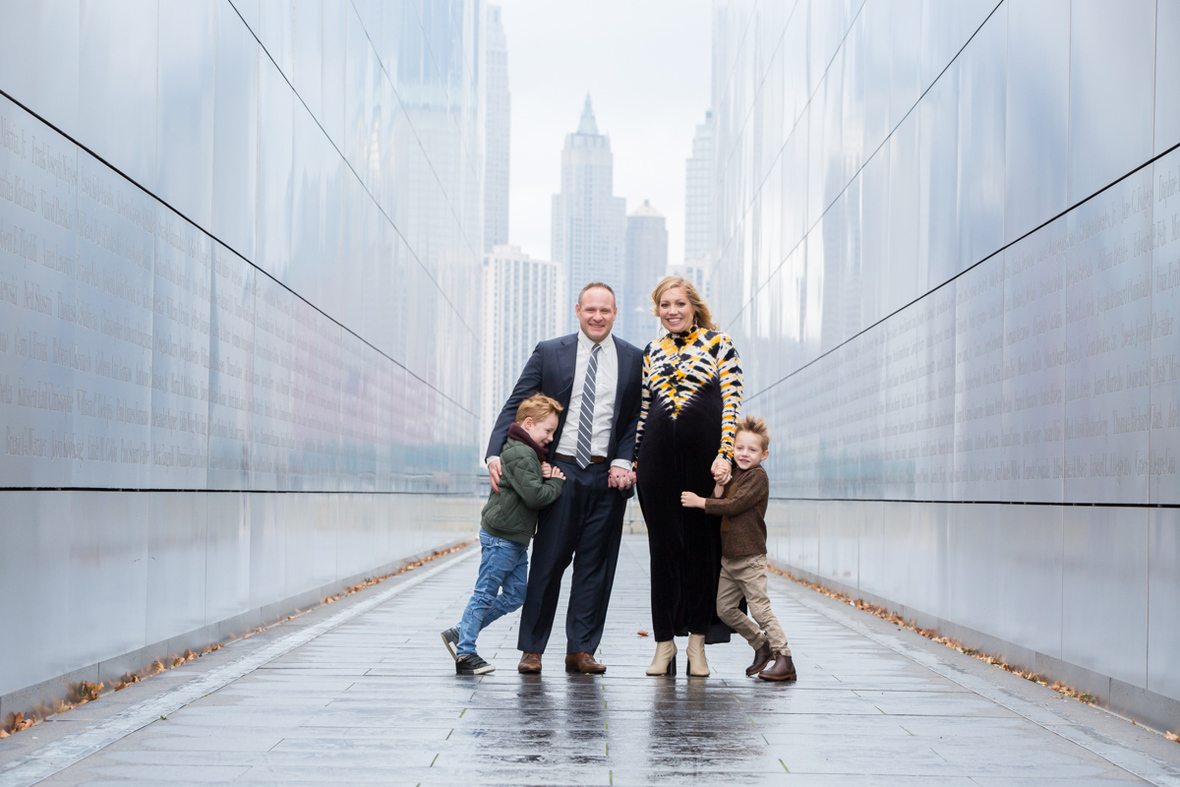Empty Sky Memorial - Liberty State Park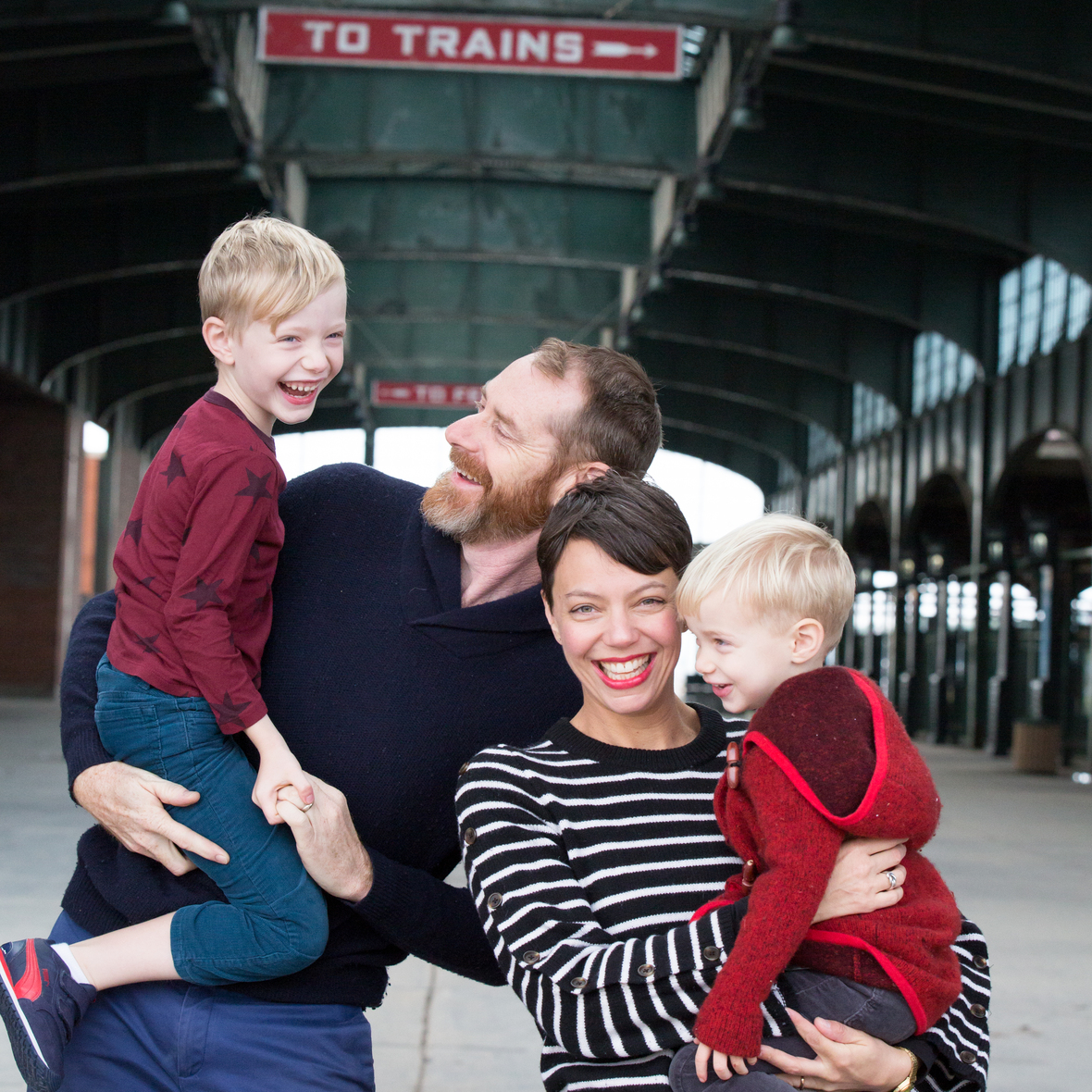Liberty State Park
 table div table+table+table+table+table+table+table+table+table+table+table+table+table+table+table+table+table+table+table+table+table+table+table div table{width:100%;padding:0}table div table+table+table+table+table+table+table+table+table+table+table+table+table+table+table+table+table+table+table+table+table+table+table div table img{width:96.23%;padding:0;float:none}table div table+table+table+table+table+table+table+table+table+table+table+table+table+table+table+table+table+table+table+table+table+table+table div table td{width:100%;padding:0 1.88% 18px}/* styles */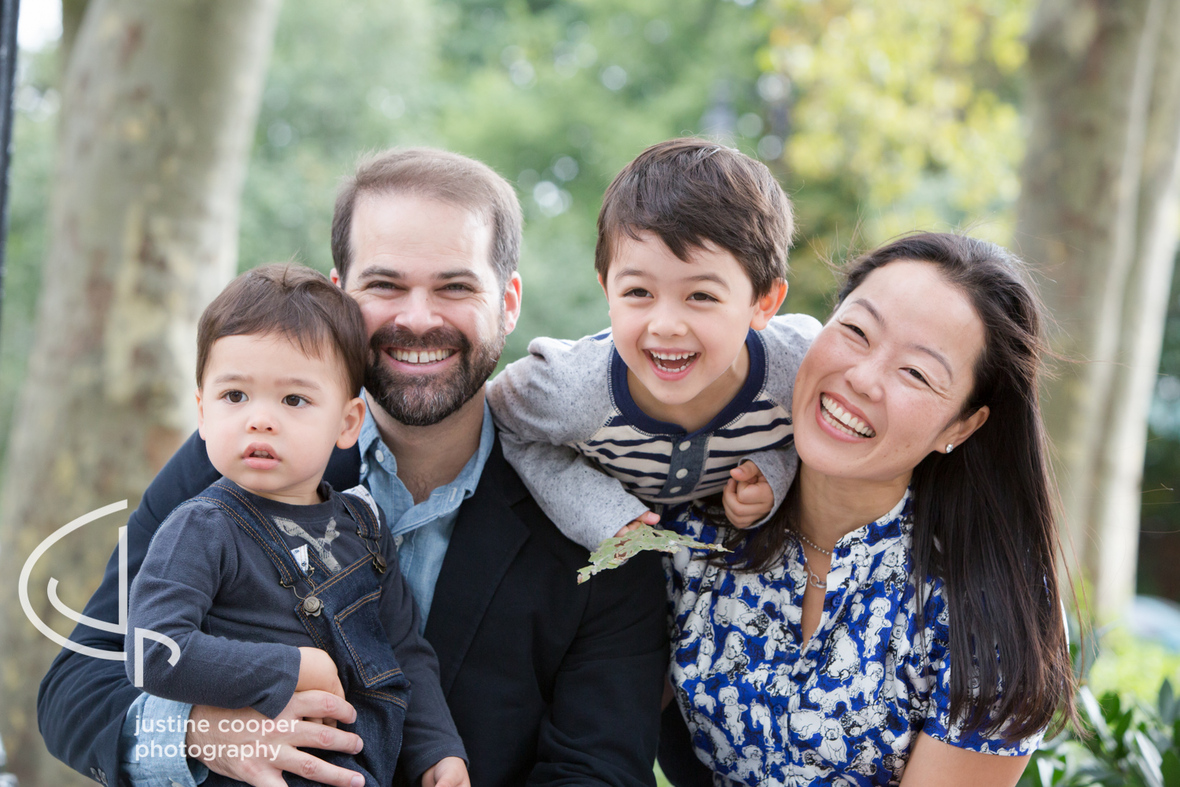Grand Army Plaza Brooklyn

Grand Army Plaza has historic architecture, foliage, and a great central fountain. All while retaining an urban feel.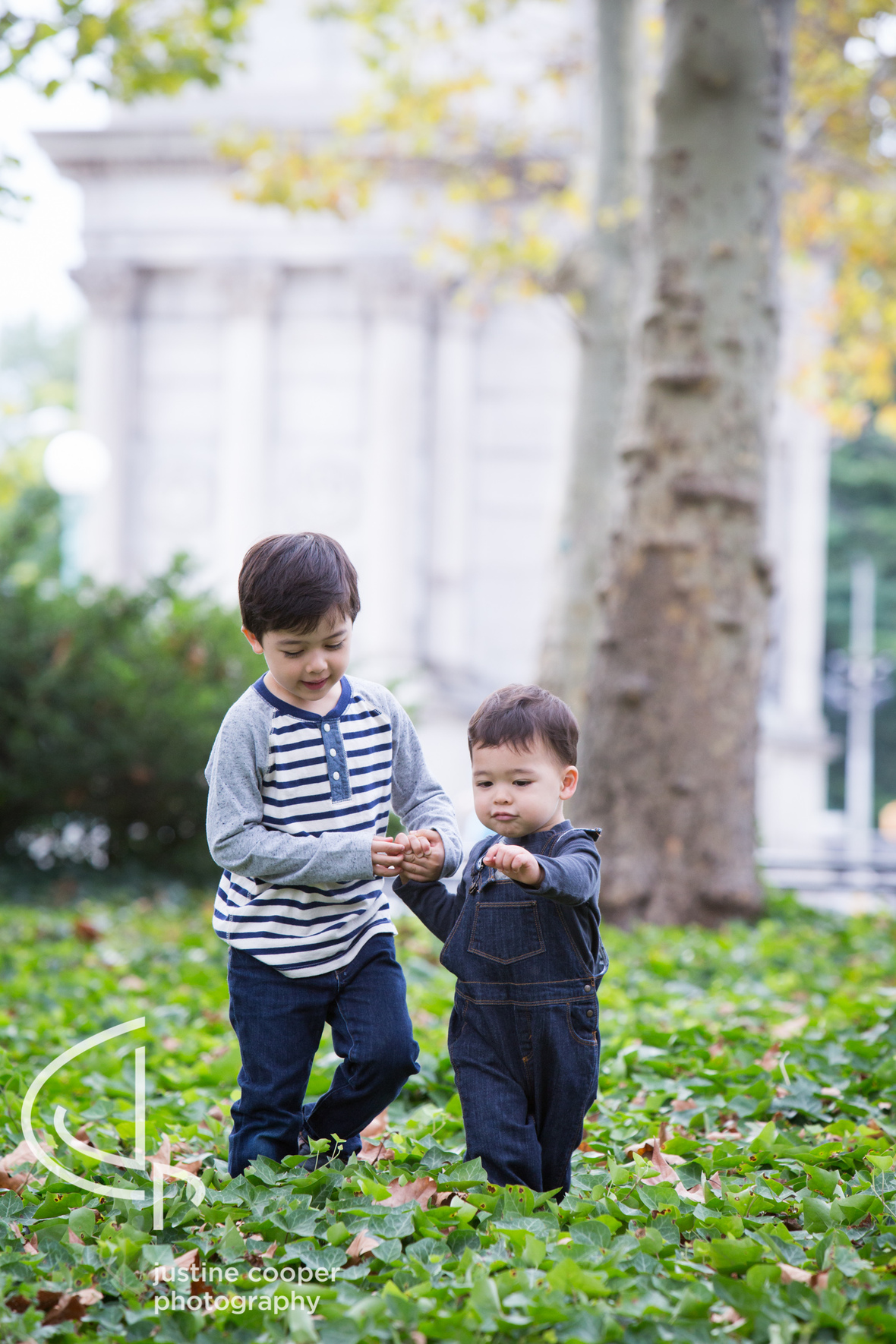Grand Army Plaza Brooklyn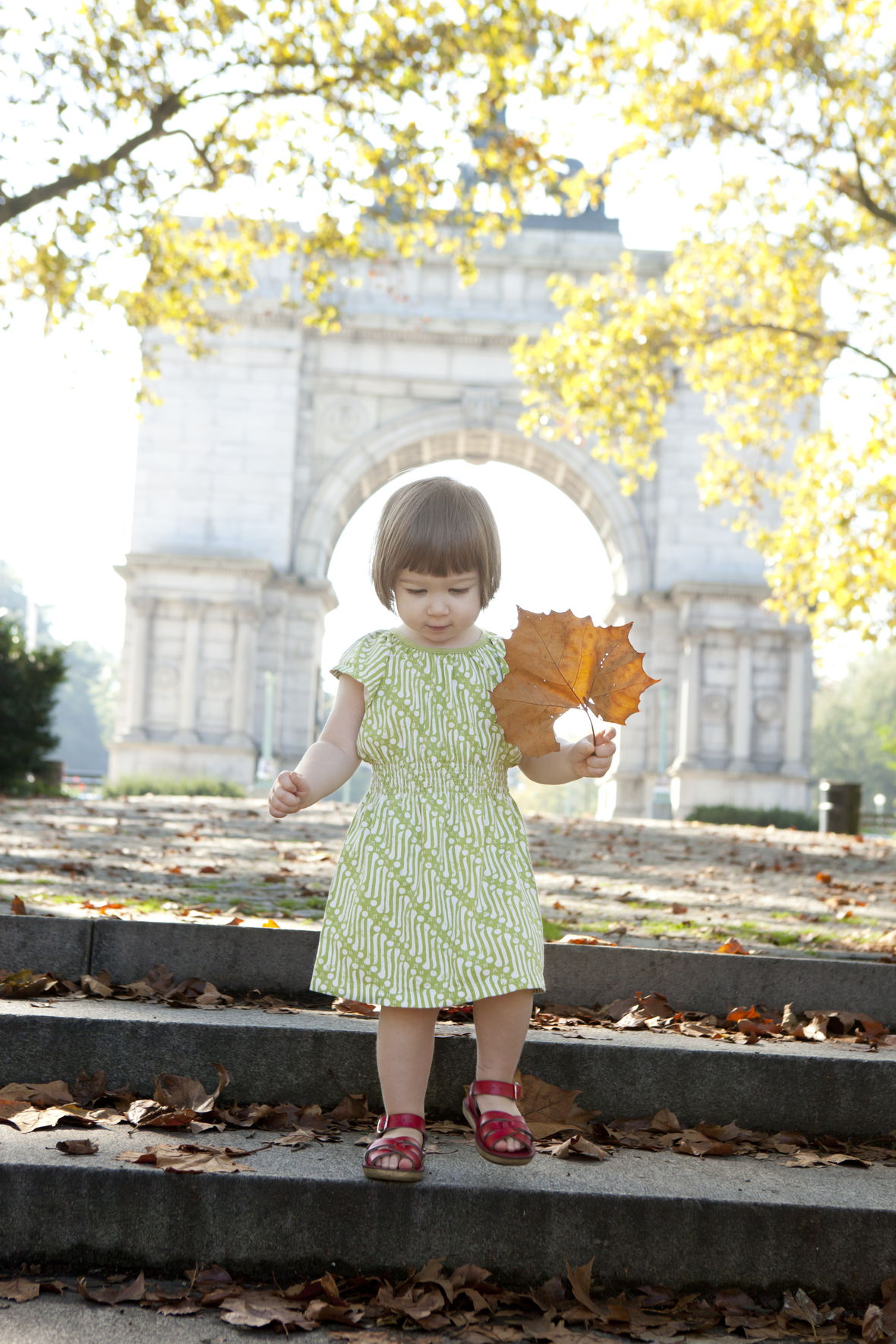Grand Army Plaza Brooklyn
 table div table+table+table+table+table+table+table+table+table+table+table+table+table+table+table+table+table+table+table+table+table+table+table+table+table+table div table{width:100%;padding:0}table div table+table+table+table+table+table+table+table+table+table+table+table+table+table+table+table+table+table+table+table+table+table+table+table+table+table div table img{width:96.23%;padding:0;float:none}table div table+table+table+table+table+table+table+table+table+table+table+table+table+table+table+table+table+table+table+table+table+table+table+table+table+table div table td{width:100%;padding:0 1.88% 18px}/* styles */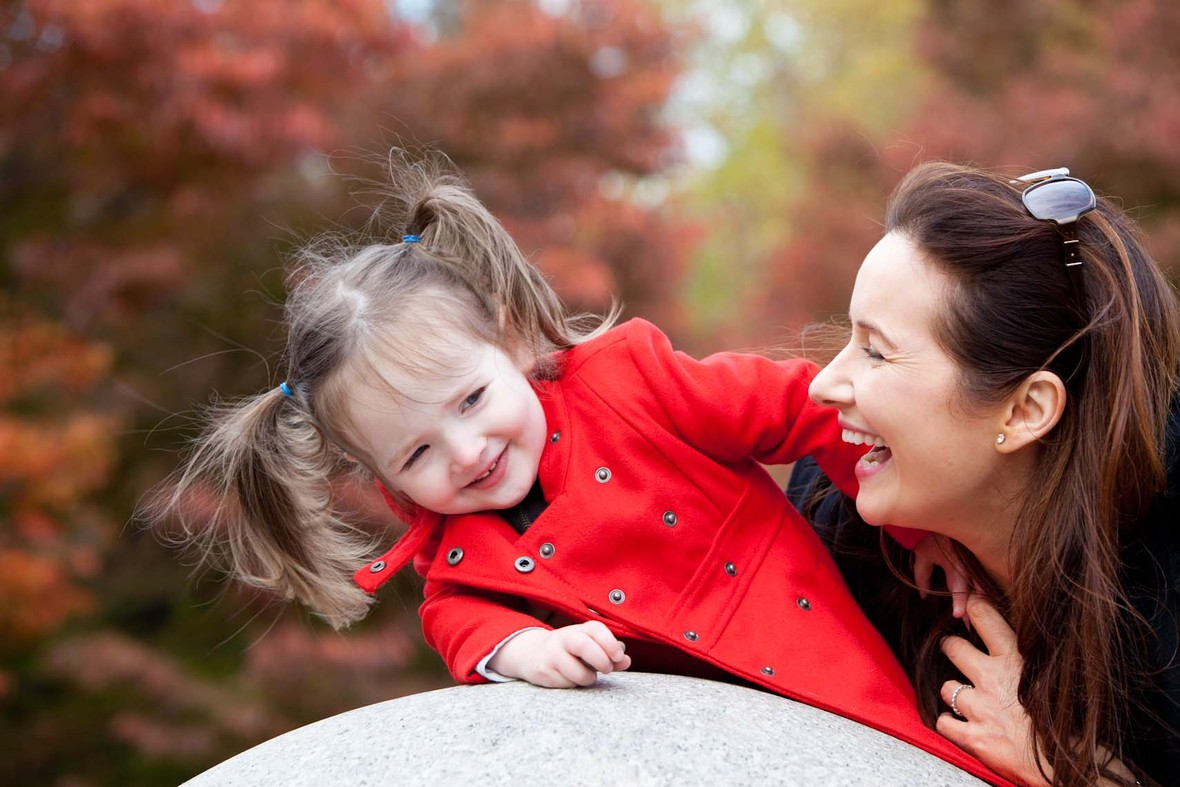Fort Greene Park

Fort Green Park offers a pretty plaza and steps, beautiful mature trees, and views to manhattan.

 table div table+table+table+table+table+table+table+table+table+table+table+table+table+table+table+table+table+table+table+table+table+table+table+table+table+table+table+table div table{width:100%;padding:0}table div table+table+table+table+table+table+table+table+table+table+table+table+table+table+table+table+table+table+table+table+table+table+table+table+table+table+table+table div table img{width:96.23%;padding:0;float:none}table div table+table+table+table+table+table+table+table+table+table+table+table+table+table+table+table+table+table+table+table+table+table+table+table+table+table+table+table div table td{width:100%;padding:0 1.88% 18px}/* styles */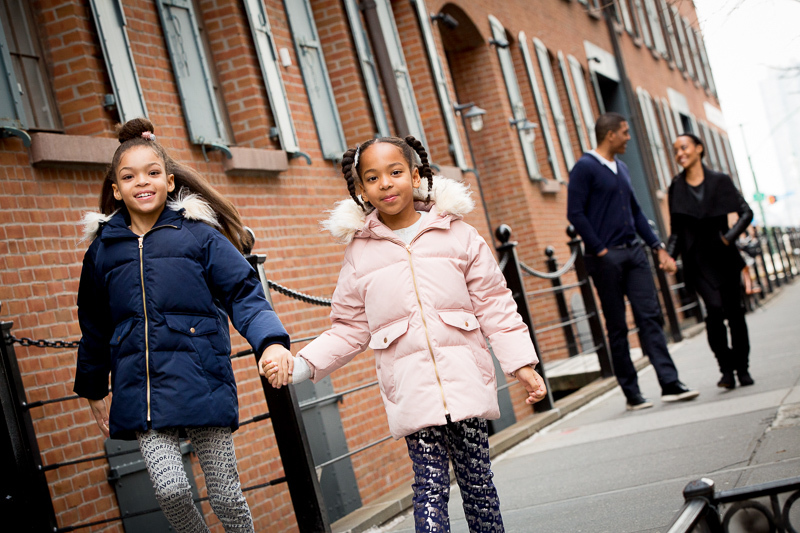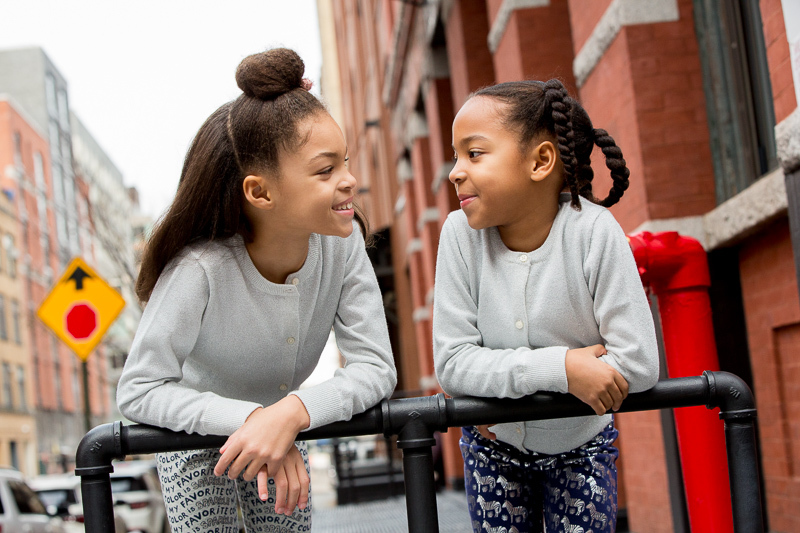Tribeca is a perrenial favorite for its beautiful 19th century loft buildings, the Staple St bridge and cobblestone streets.

 table div table+table+table+table+table+table+table+table+table+table+table+table+table+table+table+table+table+table+table+table+table+table+table+table+table+table+table+table+table+table+table div table{width:100%;padding:0}table div table+table+table+table+table+table+table+table+table+table+table+table+table+table+table+table+table+table+table+table+table+table+table+table+table+table+table+table+table+table+table div table img{width:96.23%;padding:0;float:none}table div table+table+table+table+table+table+table+table+table+table+table+table+table+table+table+table+table+table+table+table+table+table+table+table+table+table+table+table+table+table+table div table td{width:100%;padding:0 1.88% 18px}/* styles */## Can't make the date work?

 /* styles */ If you've made it this far, congratulations! If you can't make a mini session day or don't need a 2 hour session Justine has a 1 hour weekday session available. \$950 includes all the digital files (\$1050 on a weekend). Invite a friend to book one on the same day before or after your session, and each receive \$100 off. Or have a custom 90 minute session with Petra and a set of your favorite 30 digital files for \$750. studio@justinecooperphotography.com